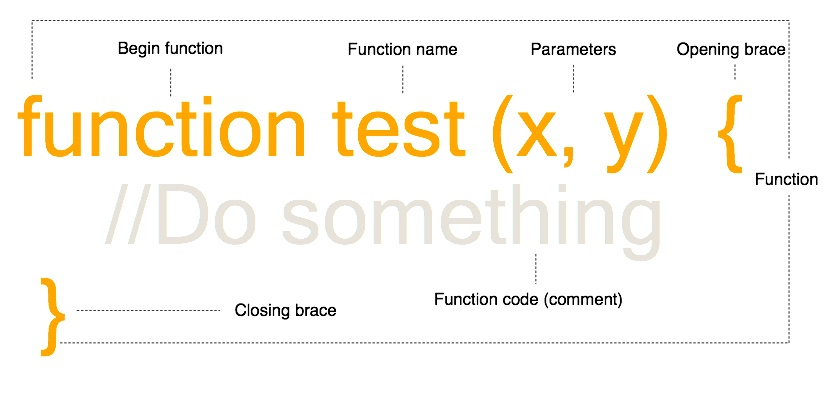# Part 3.1Functions in JavaScript

Methods play a huge role in any programming language.

## What is a function is

JavaScript can be placed entirely in one single page or it can be divided into small sub-routines or methods known as functions. Functions do not need to return anything in JavaScript (although strictly speaking they simply return a void value) meaning that a call to a function does not need to give something back.

A function represents a mathematical function machine or the IPO cycle:

Input -> Process -> Output

Methods are useful for several reasons, the first being that they can be used over and over again without needing to rewrite the code each time. The second reason is they make the code more readable and easier to understand. A final reason for this, and perhaps the most important in relation to JavaScript is that it makes it possible for a file (for example, the HTML file that the JavaScript is contained in or referenced from) other than the JavaScript file containing the code to reference a piece of code.

## Functions in JavaScript

The following is the structure of a JavaScript function:With the above function no code is actually executed as there is simply a comment inside the function.

The following sample demonstrates how to call a function:

JavaScript
function test_function () {
//Do something
console.log("Hello");
};
test_function();


### Passing parameters in JavaScript

Functions can have parameters passed to them, which are values that the function names based on their position in the function call. Assuming the function was changed to:

JavaScript
function test_function (x, y) {
//Do something
};
test_function(5, 2);


The call to the function would be able to specify two parameters, x and y as shown above.

### Returning a value

A function can also give back a value. This allows functions to do some processing and then give something as an output:

For instance:

JavaScript
function test_function (x, y) {
return 5 + x * y;
};
console.log(test_function(5, 2));


## Anonymous functions

Anonymous functions or lambda functions are a method of declaring a function without a name. These functions themselves can be assigned to a varaible or object property, but they do not have names.

An anonymous function is defined by not putting the name after the function keyword:

JavaScript
function (x, y) {
return 5 + x * y;
}


In the following sample the function is assigned to a variable and called:

JavaScript
var f = function (x, y) {
return 5 + x * y;
};
console.log(f(5, 2));


Anonymous functions can also be embedded as paramters to another function:

JavaScript
var f = function (x, y) {
//Since x is a function, it must be evaluated first.
return 5 + x() * y;
};
console.log(f(function(){ return 10; }, 2));


Notice that the first parameter is a function. This is passing an anonymous function as a parameter.

Feedback 👍
Comments are sent via email to me.